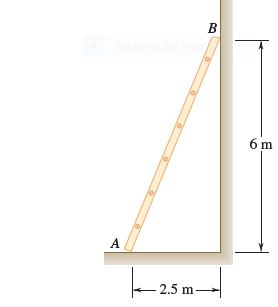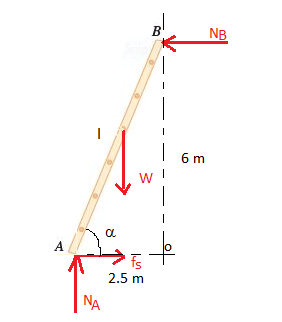# A 6.5-m ladder AB leans against a wall as shown. Assuming that the coefficient of static friction μs is zero at B, determine the smallest value of μs at A for which equilibrium is maintained

Question-AnswerCategory: Engineering MechanicsA 6.5-m ladder AB leans against a wall as shown. Assuming that the coefficient of static friction μs is zero at B, determine the smallest value of μs at A for which equilibrium is maintained

A 6.5-m ladder AB leans against a wall as shown. Assuming that the coefficient of static friction μs is zero at B, determine the smallest value of μs at A for which equilibrium is maintained.The free-body diagram of the ladder is shown belowStep 1Step 2